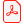# Conference article

## Internalized State-Selection: Generation and Integration of Quasi-Linear Differential-Algebraic Equations

Christoph Höger
Institute of Software Engineering and Theoretical Computer Science, TU Berlin, Germany

Andreas Steinbrecher
Department of Mathematics, TU Berlin, Germany

Download articlehttp://dx.doi.org/10.3384/ecp1511899

Linköping Electronic Conference Proceedings 118:10, s. 99-107

Published: 2015-09-18

ISBN: 978-91-7685-955-1

ISSN: 1650-3686 (print), 1650-3740 (online)

### Abstract

In modeling and simulation of dynamical processes frequently higher index differential-algebraic equations (DAEs) arise. Since an attempt to solve higher-index DAEs directly yields several numerical problems, a regularization in combination with a robust and efficient integration is required. \qualidaes\ is a DAE solver designed to make explicit use of such a regularization. It allows for the solution of over-determined quasi-linear DAEs of the form $M(x,t)\dot{x}=f(x,t)$, $0=g(x,t)$. Such DAEs arise naturally if a quasi-linear DAE is regularized by augmentation with the set of its (hidden) constraints. General DAEs can be brought into the quasi-linear form. To this end, \modelica\ equations can be transformed into the specific input format expected by \qualidaes. This transformation can be implemented in a functional style and yields a non-trivial result. Additionally it provides an on-the-fly solution for the occurrence of higher-order derivatives.

### Keywords

Differential-Algebraic Equations; Quasi-Linear; Modelica; Translation; Regularization; Solver; QUALIDAES

### References

R. Altmeyer and A. Steinbrecher. Regularization and numerical simulation of dynamical systems modeled with Modelica. Preprint 29-2013, Institut für Mathematik, TU Berlin, 2013.

K.E. Brenan, S.L. Campbell, and L.R. Petzold. Numerical Solution of Initial-Value Problems in Differential Algebraic Equations, volume 14 of Classics in Applied Mathematics. SIAM, Philadelphia, PA, 1996.

P. Deuflhard. Newton methods for nonlinear problems. Affine invariance and adaptive algorithms, volume 35 of Springer Series in Computational Mathematics. Springer-Verlag, Berlin, 2004.

C.W. Gear. Differential-algebraic equation index transformations. SIAM Journal on Scientific and Statistic Computing, 9:39–47, 1988.

E. Griepentrog and R. März. Differential-Algebraic Equations and Their Numerical Treatment, volume 88 of Teubner-Texte zur Mathematik. BSB B.G.Teubner Verlagsgesellschaft, Leipzig, 1986.

E. Hairer and G. Wanner. Solving Ordinary Differential Equations II - Stiff and Differential-Algebraic Problems. Springer-Verlag, Berlin, Germany, 2nd edition, 1996.

D.J. Higham and N.J. Higham. MATLAB Guide. Society for Industrial and Applied Mathematics (SIAM), Philadelphia, PA, second edition, 2005. ISBN 0-89871-578-4.

C. Höger. Operational semantics for a modular equation language. AVICPS 2013, page 5, 2013.

C. Höger. Dynamic structural analysis for daes. In Proceedings of the 2014 Summer Simulation Multiconference, page 12. Society for Computer Simulation International, 2014.

P. Kunkel and V. Mehrmann. Index reduction for differentialalgebraic equations by minimal extension. Zeitschrift für Angewandte Mathematik und Mechanik, 84(9):579–597, 2004.

P. Kunkel and V. Mehrmann. Differential-Algebraic Equations. Analysis and Numerical Solution. EMS Publishing House, Zürich, Switzerland, 2006.

S. Mattsson and G. Söderlind. Index reduction in differentialalgebraic equations using dummy derivatives. SIAM Journal on Scientific and Statistic Computing, 14:677–692, 1993.

C.C. Pantelides. The consistent initialization of differentialalgebraic systems. SIAM Journal on Scientific and Statistic Computing, 9:213–231, 1988.

P. Pepper, A. Mehlhase, Ch. Höger, and L. Scholz. A compositional semantics for Modelica-style variable-structure modeling. In P. Fritzson F.E. Cellier, D. Broman and E.A. Lee, editors, 4th International Workshop on Equation-Based Object-oriented Modeling Languages and Tools (EOOLT 2011), number 56 in Linköping Electronic Conference Proceedings, pages 45–54, Zurich, Switzerland, 2011. September 5, 2011.

J. Pryce. A simple structural analysis method for DAEs. BIT Numerical Mathematics, 41:364–394, 2001.

L. Scholz and A. Steinbrecher. A combined structuralalgebraic approach for the regularization of coupled systems of DAEs. Preprint 30-2013, Institut für Mathematik, TU Berlin, 2013.

L. Scholz and A. Steinbrecher. Efficient numerical integration of dynamical systems based on structural-algebraic regularization avoiding state selection. In K.-E. Arzen H. Tummescheit, editor, Proceedings of the 10th International Modelica Conference, March 10-12, 2014, Lund, Sweden, number 96 in Linköping Electronic Conference Proceedings, pages 1171–1178. Modelica Association and Linköping University Electronic Press, 2014.

A. Steinbrecher. Numerical Solution of Quasi-Linear Differential-Algebraic Equations and Industrial Simulation of Multibody Systems. PhD thesis, Technische Universität Berlin, 2006.